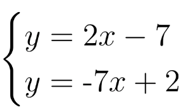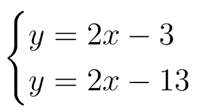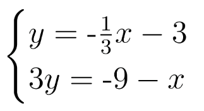# A.2.17.3 Sorting Systems

Determine if the system below has: (1) no solution, (2) one solution or (3) infinitely many solutions and then explain how you know.Determine if the system below has: (1) no solution, (2) one solution or (3) infinitely many solutions and then explain how you know.Determine if the system below has: (1) no solution, (2) one solution or (3) infinitely many solutions and then explain how you know.